Related Articles

# Violin plot using Plotly in Python

• Last Updated : 16 Mar, 2021

Violin Plot is a method to visualize the distribution of numerical data of different variables. It is similar to Box Plot but with a rotated plot on each side, giving more information about the density estimate on the y-axis. The density is mirrored and flipped over and the resulting shape is filled in, creating an image resembling a violin. The advantage of a violin plot is that it can show nuances in the distribution that aren’t perceptible in a boxplot. On the other hand, the boxplot more clearly shows the outliers in the data.

Violin Plots hold more information than the box plots, they are less popular. Because of their unpopularity, their meaning can be harder to grasp for many readers not familiar with the violin plot representation.

Plotly is an open-source python module that is a very powerful data visualization tool. It supports various plots to represent and study data easily. This article discusses how a violin plot can be obtained using Plotly with the help of its two classes namely- express and graph_objects.

The classes can be chosen as per one’s convenience, but the approach remains the same.

Approach:

• Import module
• Import data
• Call violinplot with required parameters
• Display plot

Function supported by Plotly.express

Syntax:

violin(data_frame=None, x=None, y=None, color=None, facet_row=None, facet_col=None, facet_col_wrap=0, facet_row_spacing=None, facet_col_spacing=None, hover_name=None, hover_data=None, custom_data=None, animation_frame=None, animation_group=None, category_orders=None, labels=None, color_discrete_sequence=None, color_discrete_map=None, orientation=None, violinmode=None, log_x=False, log_y=False, range_x=None, range_y=None, points=None, box=False, title=None, template=None, width=None, height=None)

Function supported by Plotly.graph_objects

Syntax:

Violin(arg, alignmentgroup, bandwidth, box_visible, customdata, customdatasrc, fillcolor, hoverinfo, hoverinfosrc, hoverlabel, hoveron, hovertemplate, hovertemplatesrc, hovertext, hovertextsrc, ids, idssrc, jitter, legendgroup, line, marker, meanline, meta, metasrc, name, offsetgroup, opacity, orientation, pointpos, points, scalegroup, scalemode, selected, selectedpoints, showlegend, side, span, spanmode, stream, text, textsrc, uid, uirevision, unselected, visible, width, x, x0, xaxis, xsrc, y, y0, yaxis, ysrc, **kwargs)

Data in use: Bestsellers

### Approach

• Import module
• Plot using violin()
• Display plot

Example 1: Using Plotly.express

## Python3

 `import` `plotly.express as pt ` `import` `pandas as pd ` ` `  `data ``=` `pd.read_csv(``"C:\\Users\\Vanshi\\Desktop\\gfg\\bestsellers.csv"``) ` `df ``=` `pd.DataFrame(data) ` `data ``=` `df.head() ` ` `  `fig ``=` `pt.violin(data, y``=``"Year"``) ` `fig.show() `

Output: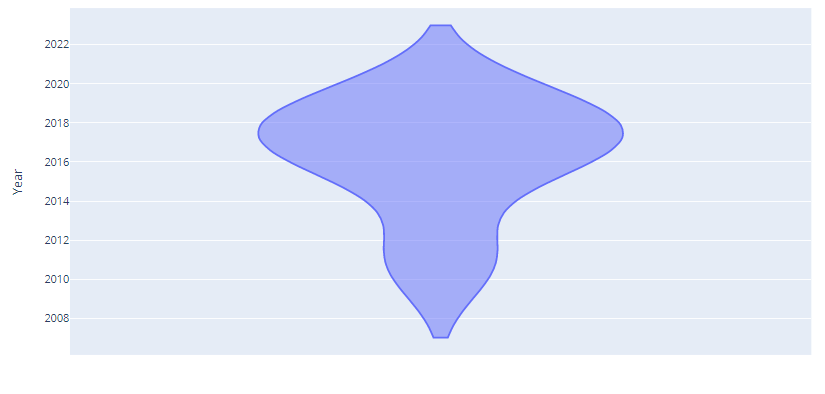Example 2: Using Graph_objects

## Python3

 `import` `plotly.graph_objects as go ` `import` `pandas as pd ` ` `  `data ``=` `pd.read_csv(``"C:\\Users\\Vanshi\\Desktop\\gfg\\bestsellers.csv"``) ` `df ``=` `pd.DataFrame(data) ` ` `  `plot ``=` `go.Figure(data``=``go.Violin(y``=``df[``'Price'``])) ` `plot.show() `

Output: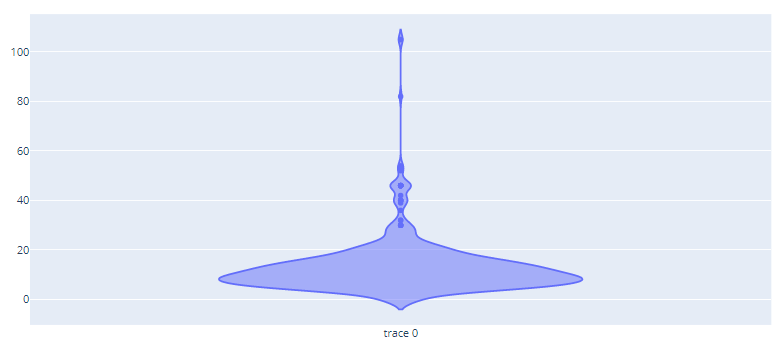## Violin plot with box and scatter plot

The above examples depict a simple violin plot but it can be visualized along with other visualization depict within the same frame. The given example shows how a violin plot can be drawn along with box plots and scatter plot.

Example 1: Using Plotly.express

## Python3

 `import` `plotly.express as pt ` `import` `pandas as pd ` ` `  `data ``=` `pd.read_csv(``"C:\\Users\\Vanshi\\Desktop\\gfg\\bestsellers.csv"``) ` `df ``=` `pd.DataFrame(data) ` `data ``=` `df.head() ` ` `  `# display box and scatter plot along with violin plot ` `fig ``=` `pt.violin(data, y``=``"Year"``, box``=``True``, points``=``'all'``) ` ` `  `fig.show() `

Output: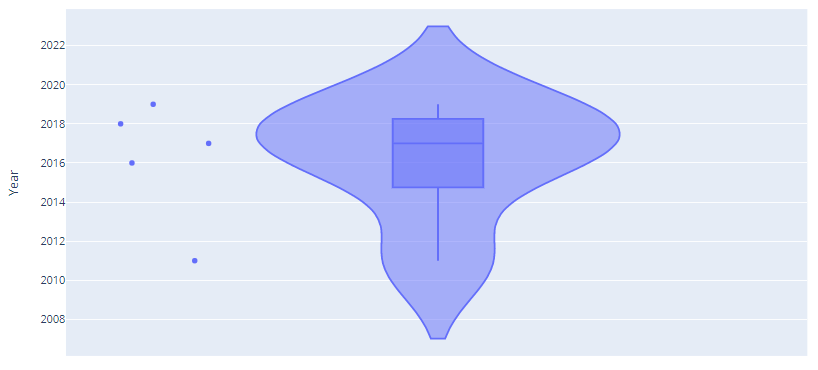Example 2: Using graph_objects

## Python3

 `import` `plotly.graph_objects as go ` `import` `pandas as pd ` ` `  `data ``=` `pd.read_csv(``"C:\\Users\\Vanshi\\Desktop\\gfg\\bestsellers.csv"``) ` `df ``=` `pd.DataFrame(data) ` ` `  `plot ``=` `go.Figure(data``=``go.Violin( ` `    ``y``=``df[``'Price'``], points``=``'all'``, pointpos``=``2``, box_visible``=``True``)) ` ` `  `plot.show() `

Output: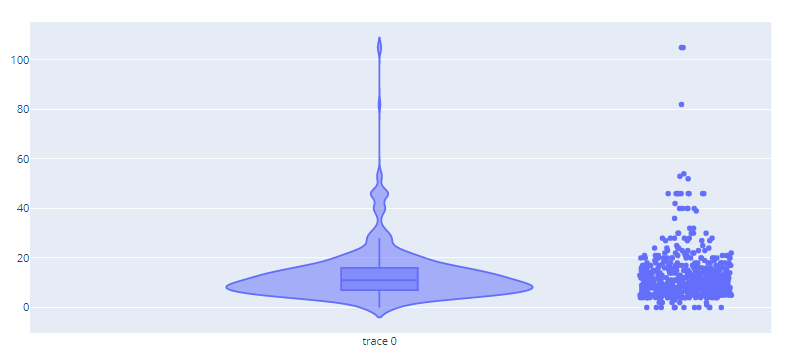## Multiple violin plot

It is possible using plotly to visualize multiple violin plots in one frame to compare them simultaneously.

Example 1: Using Plotly express

## Python3

 `import` `plotly.express as px ` `import` `pandas as pd ` ` `  `data ``=` `pd.read_csv(``"C:\\Users\\Vanshi\\Desktop\\gfg\\bestsellers.csv"``) ` `df ``=` `pd.DataFrame(data) ` ` `  `plot ``=` `px.violin(x``=``df[``'Year'``], y``=``df[``'Price'``]) ` `plot.show() `

Output: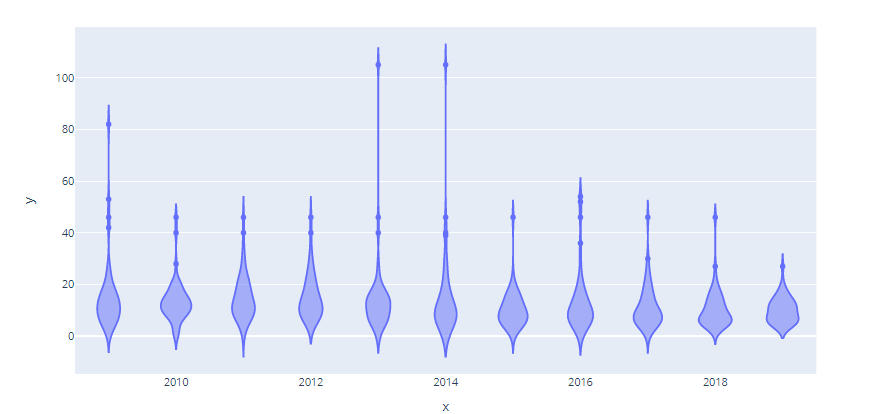Example 2: Using graph_objects

## Python3

 `import` `plotly.graph_objects as go ` `import` `pandas as pd ` ` `  `data ``=` `pd.read_csv(``"C:\\Users\\Vanshi\\Desktop\\gfg\\bestsellers.csv"``) ` `df ``=` `pd.DataFrame(data) ` ` `  `plot ``=` `go.Figure(data``=``go.Violin(x``=``df[``'Year'``], y``=``df[``'Price'``])) ` `plot.show() `

Output: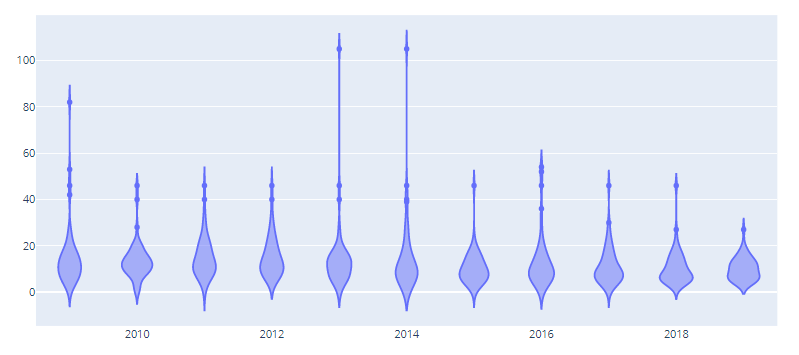## Grouped violin plot

A grouped violin plot allow comparison of multiple violin plot but which have something in common i.e. it allows comparison of two contemporary violin plots

Example 1: Using Plotly express

## Python3

 `import` `plotly.express as px ` `import` `pandas as pd ` ` `  `data ``=` `pd.read_csv(``"C:\\Users\\Vanshi\\Desktop\\gfg\\bestsellers.csv"``) ` `df ``=` `pd.DataFrame(data) ` ` `  `plot ``=` `px.violin(df, x``=``df[``'Year'``], y``=``df[``'Price'``], color``=``df[``'Genre'``]) ` `plot.show() `

Output: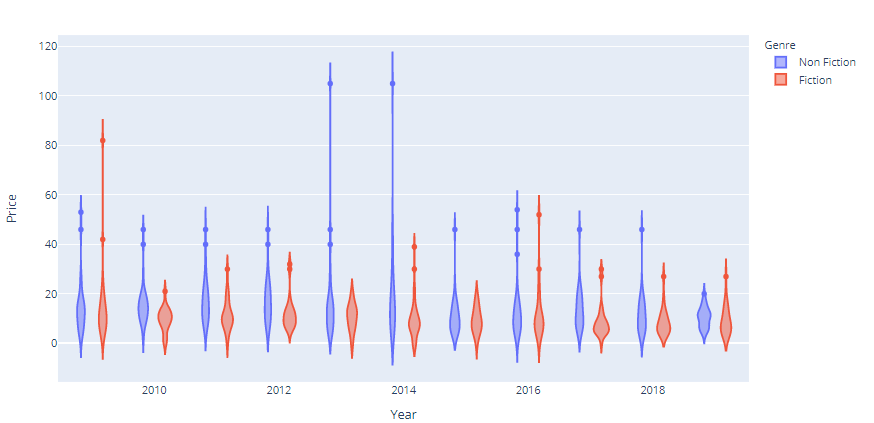Example 2: Using graph_objects

## Python3

 `import` `plotly.graph_objects as go ` `import` `pandas as pd ` ` `  `data ``=` `pd.read_csv(``"C:\\Users\\Vanshi\\Desktop\\gfg\\bestsellers.csv"``) ` `df ``=` `pd.DataFrame(data) ` ` `  `plot ``=` `go.Figure() ` ` `  `plot.add_trace(go.Violin(x``=``df[``'Year'``][df[``'Genre'``] ``=``=` `'Fiction'``], ` `                         ``y``=``df[``'Price'``], line_color``=``'red'``, name``=``'Fiction'``)) ` ` `  `plot.add_trace(go.Violin(x``=``df[``'Year'``][df[``'Genre'``] ``=``=` `'Non Fiction'``], ` `                         ``y``=``df[``'Price'``], line_color``=``'blue'``, name``=``'Non-Fiction'``)) ` ` `  `plot.update_layout(violinmode``=``'group'``) ` `plot.show() `

Output: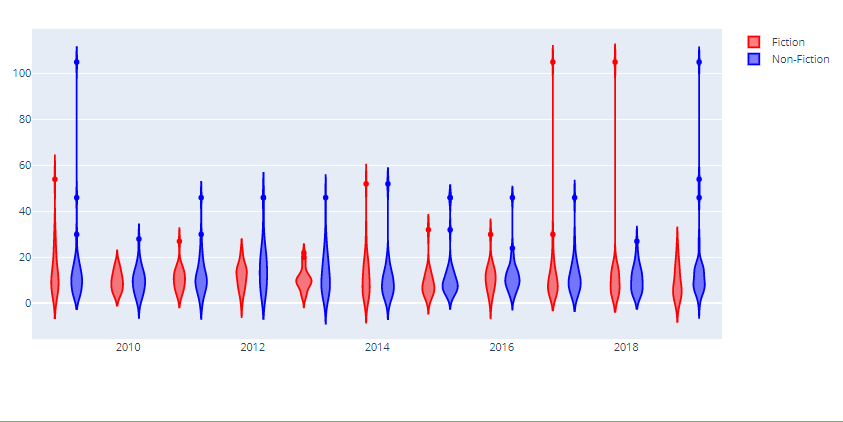Attention geek! Strengthen your foundations with the Python Programming Foundation Course and learn the basics.

To begin with, your interview preparations Enhance your Data Structures concepts with the Python DS Course. And to begin with your Machine Learning Journey, join the Machine Learning – Basic Level Course

My Personal Notes arrow_drop_up
Recommended Articles
Page :